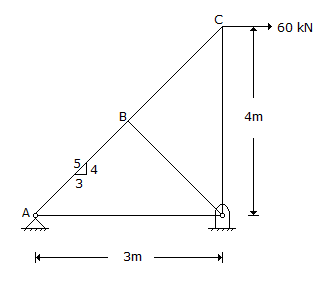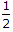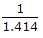# Civil Engineering - UPSC Civil Service Exam Questions

21.

Due to horizontal pull of 60 kN at C, what is the force induced in the member AB?A. 0 B. 40 kN C. 80 kN D. 100 kN

Explanation:

No answer description available for this question. Let us discuss.

22.

California Bearing Ratio (CBR) is a :

 A. measure of soil strength B. method of soil identification C. measure to indicate the relative strengths of paving materials D. measure of shear strength under lateral confinement

Explanation:

No answer description available for this question. Let us discuss.

23.

Consider the following statements :
1. Masonry in rich cement mortar though having good strength with high shrinkage is much liable for surface cracks.
2. Lime mortar possesses poor workability and poor water retentivity and also suffers high shrinkage.
3. Masonry in lime mortar has better resistance against rain penetration and is less liable to crack when compared to masonry in cement mortar.
Which of these statements are correct ?

 A. 1, 2 and 3 B. 1 and 2 C. 2 and 3 D. 1 and 3

Explanation:

No answer description available for this question. Let us discuss.

24.

For a given stress, the ratio of the moment of resistance of a beam of square section when placed with one diagonal horizontal to the moment of resistance of the same beam when placed with two sides horizontal will be

 A.B. 2 C. 1.414 D.Explanation:

No answer description available for this question. Let us discuss.

25.

A water jet 0.02 m2 in area has a velocity of 15 m/s. If the jet impinges normally on a plate which is moving at a velocity 5 m/s in the direction of the jet, what is the force on the plate due to this impact ? (Density = 1000 kg/m3)

 A. 200 N B. 2000 N C. 20000 N D. 4000 N